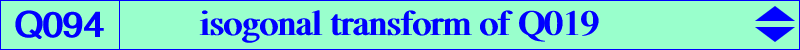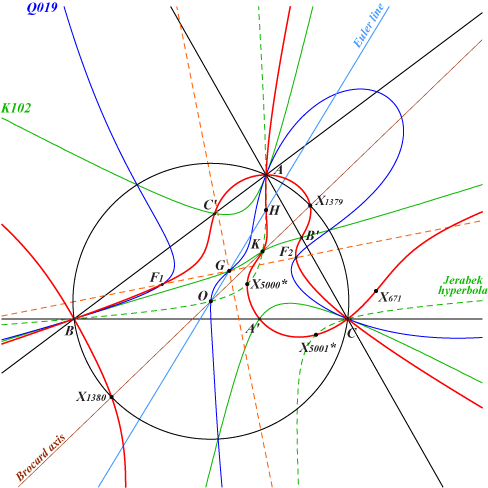too complicated to be written here. Click on the link to download a text file.X(4), X(6), X(671), X(1379), X(1380) isogonal conjugates of X(5000), X(5001) four foci of the Steiner in-ellipse (two real F1, F2) infinite points of the Grebe cubic K102 A'B'C' cevian triangle of K imaginary foci of the Brocard ellipse i.e. common points of the Brocard axis and the Kiepert hyperbola anti-points, see Table 77Q094 is the isogonal transform of the quartic Q019. These two curves are related to {X}-Pedal and {X}-Antipedal points as in Table 45. More precisely, for any strong center X on the Grebe cubic K102, the {X}-Pedal points lie on Q094. For example, the {X(6)}-Pedal points are the four foci of the Steiner in-ellipse. Q094 is a circular quintic with focus the Lemoine point K. The three other asymptotes are parallel to those of the Grebe cubic K102. A, B, C are nodes and the tangents at A are AK and the parallel to BC. Q094 is the locus of point M such that K, M and the isogonal conjugate of M with respect to the circumcevian triangle of M are collinear. See a generalization in the page Q050.